# 在 MetaTrader 5 中使用 MATLAB 2018 的计算功能

13 五月 2019, 08:37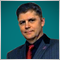0
750

### 简介

A. Emelyanov 所写文章第一章中所描述的简单和复杂的数据类型不会被改变。为了不重复定性陈述的材料，最好自己熟悉此处所述的描述。差异是在从MATLAB环境创建共享C++库的阶段出现的。

1. 利用预先编制的一组 MATLAB 模块，建立了基于季节自回归综合移动平均值>模型的C++共享库。
2. 然后把计算模块包含在MQL5程序中。为此，还创建了一个中间库，它解决了MQL5（用C/C++风格组织的存储器）和MATLAB（以矩阵形式组织的存储器）之间传输数据的问题。
3. 描述了一个预测模型，并将其嵌入到所创建的指标中，并对其性能进行了验证。

### 1. 利用 MATLAB 编译器 SDK 从 MATLAB 函数中创建共享C++库

2015年，在 MATLAB 中创建 DLL 库的过程发生了变化。至于与 MQL5 程序的集成，问题归结为 MATLAB 编译器不再用于创建库，而是面向生成自主可执行文件。自2015年以来，用于创建 DLL 库的函数已转到 MATLAB 编译器SDK。MATLAB 编译器 SDK 扩展了 MATLAB 编译器的功能，允许它从 MATLAB 程序中创建C/C++共享库、Microsoft .NET程序集和Java类。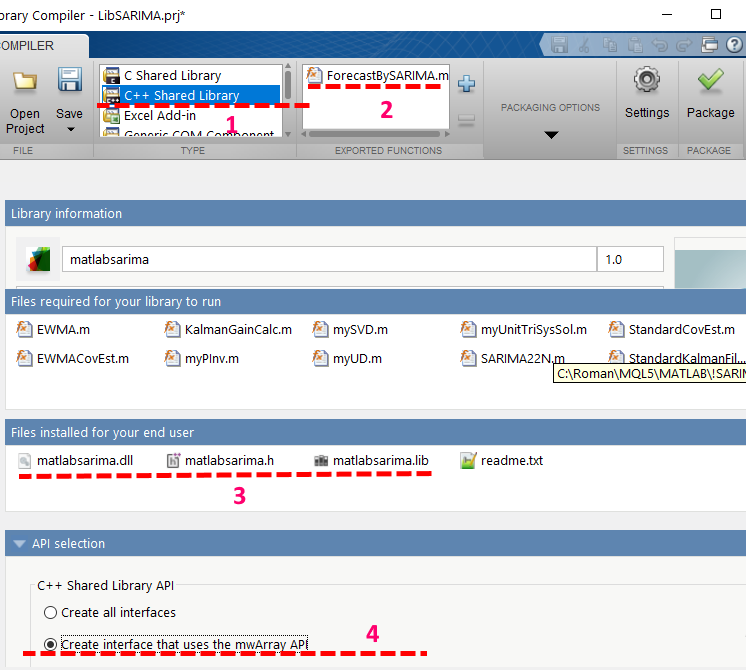1. 创建C++标准的共享库
2. ForecastSARIMA.m中显示的函数被导出，而其他函数不受导出的影响，也不被授予访问外部程序的权限。
3. 生成标准接口中用于链接的文件（matlabsarima.dll、matlabsarima.h、matlabsarima.lib）。
4. mwArray结构上采用了 MATLAB 矩阵访问接口。

### 2. 在微软 Visual C++ 中创建中介库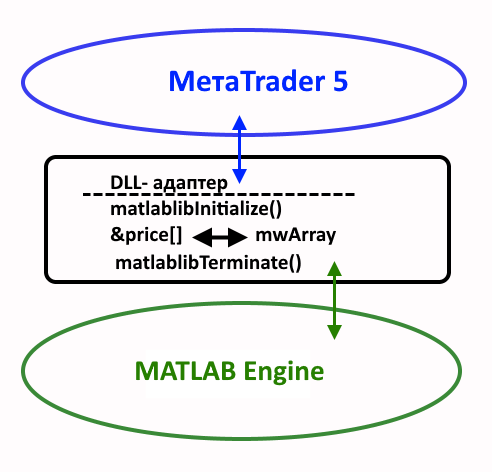2017的一个重要创新是引入了这样的结构：把 mwArray API 引入到 MATLAB，它允许将打包的函数创建和集成到C++应用程序中。之前使用的 mxArray 升级到了新的矩阵接口。还有一个集成共享库的选项 — MATLAB Data API 接口, 但是它与我们的情况不相关。

1. 第一个变量 — MATLAB_2018 应指向装有 MATLAB 或 MATLAB 运行时的目录；
2. 第二个变量 — MATLAB_2018_LIB64 应指向包含外部库的目录: <MATLAB_2018>\extern\lib\win64;
3. 第三个变量 — MATLIB_USER 应该指向放置原始库的目录。为了解决搜索原始用户库的问题，还必须将此目录添加到系统变量“Path”中。

### 2.1在Visual Studio 2017中编写适配器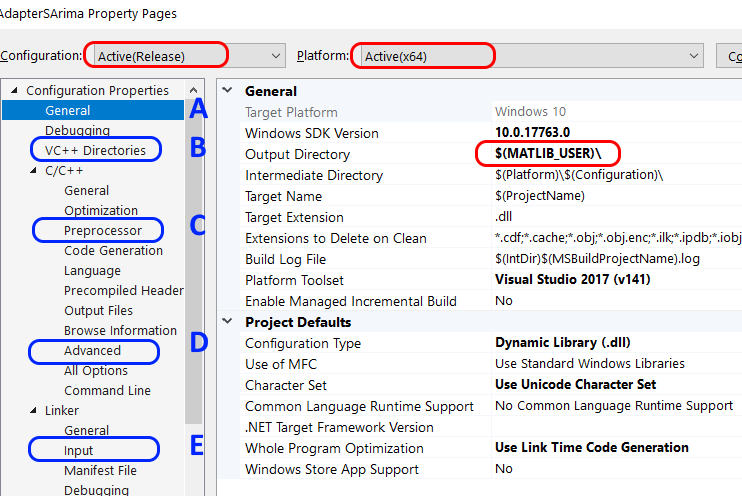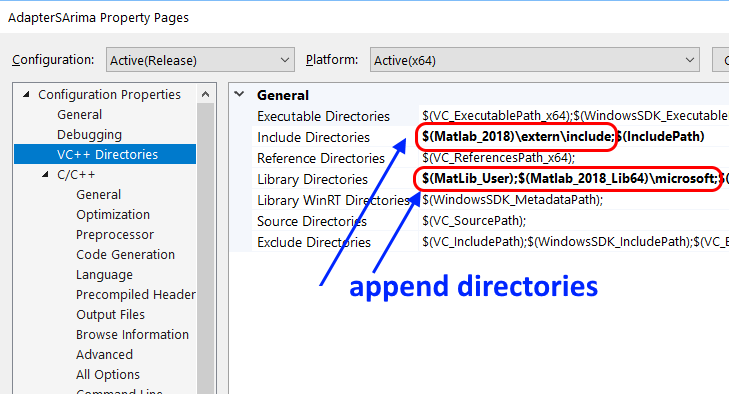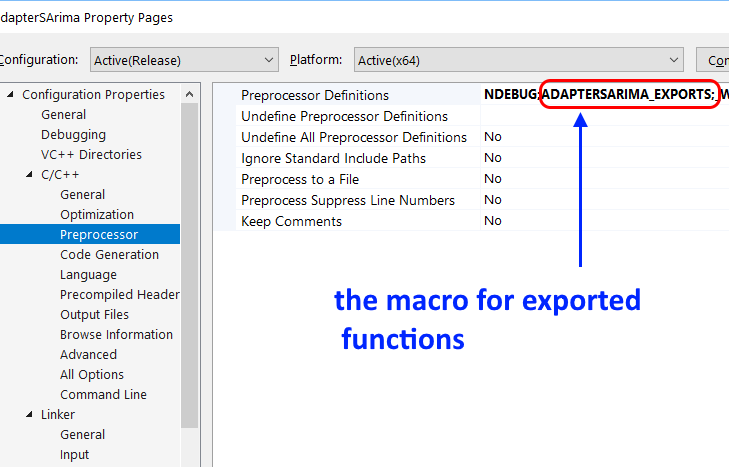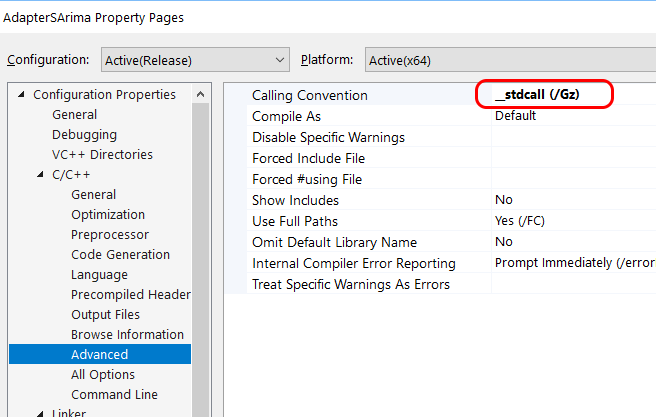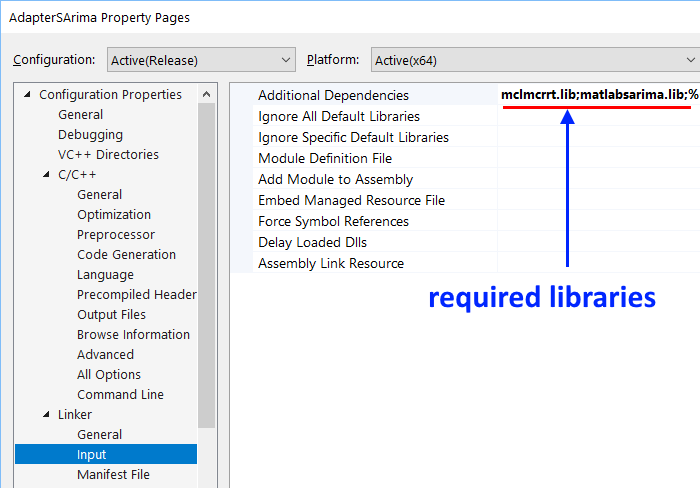1. 指定具有所需头文件的目录；
2. 指定包含所需库文件的目录；
3. 设置预处理器定义-宏，其功能在下面会探讨；
4. 指定工作所需的特定库（由 MATLAB 编写）。

1. AdapterSArima.h 文件

```#pragma once
#ifdef ADAPTERSARIMA_EXPORTS
#define _DLLAPI extern "C" __declspec(dllexport)  // 此定义对于将DLL和LIB库配对是必需的
#else
#define _DLLAPI extern "C" __declspec(dllimport)  // 绑定DLL库需要此定义
#endif
_DLLAPI int prepareSARIMA(void);
_DLLAPI int goSarima(double *Res, double *DataArray, int idx0, int nLoad, int iSeasonPriod = 28, int npredict = 25, int filterOn = 1, int PlotOn = 0);
_DLLAPI void closeSARIMA(void);
```

adaptersarima.h文件使用设置中指定的宏，来指出prepareSARIMA(), closeSARIMA() and goSarima(...) 是可以用于绑定外部程序的。

2. GoSArima.cpp 文件

```#pragma once
#include "stdafx.h"
#include "matlabsarima.h"
#include "AdapterSArima.h"

bool  SArimaStarted = false;
bool  MLBEngineStarted = false;

//-----------------------------------------------------------------------------------
_DLLAPI int prepareSARIMA(void)
{
if (!MLBEngineStarted)
{
MLBEngineStarted = mclInitializeApplication(nullptr, 0);
if (!MLBEngineStarted)
{
std::cerr << "无法初始化 Matlab Runtime (MCR)" << std::endl;
return 0;
}
}
if (!SArimaStarted)
{
try
{
SArimaStarted = matlabsarimaInitialize();
if (!SArimaStarted)
{
std::cerr << "无法初始化库属性" << std::endl;
return false;
}
return (SArimaStarted)?1:0;
}
catch (const mwException &e)
{
std::cerr << e.what() << std::endl;
return -2;
}
catch (...)
{
std::cerr << "抛出未知错误" << std::endl;
return -3;
}
}
return 1;
}
//-----------------------------------------------------------------------------------

_DLLAPI void closeSARIMA(void)
{
// 调用应用程序和库终止例程
//if (SArimaStarted)
{
matlabsarimaTerminate();
SArimaStarted = false;
}
}
//-----------------------------------------------------------------------------------

_DLLAPI int goSarima(double *Res, double *DataSeries, int idx0, int nLoad, int iSeasonPeriod, int npredict, int filterOn, int PlotOn)
{       //
// 必须提前为结果分配内存，同时考虑指标中的预测。
// 用于 Res[] 的内存必须分配. Length = nLoad+npredict !!!
//
if (!SArimaStarted)
{
SArimaStarted = (prepareSARIMA() > 0) ? true : false;
}

mwArray nSeries(1, 1, mxDOUBLE_CLASS), TimeHor(1, 1, mxDOUBLE_CLASS), MAlen(1, 1, mxDOUBLE_CLASS);
mwArray SeasonLag(1, 1, mxDOUBLE_CLASS), DoPlot(1, 1, mxDOUBLE_CLASS), DoFilter(1, 1, mxDOUBLE_CLASS);

if (SArimaStarted)
{
try
{
MAlen  = 20;         // MA 平均窗口
DoFilter  = (filterOn != 0) ? 1 : 0;
TimeHor   = npredict; // 预测点
SeasonLag = iSeasonPeriod;     // SARIMA 模型的季节性周期

DoPlot = PlotOn;    // 测试模式的绘图

nSeries = nLoad;    // 数据片段的长度 nLoad

mwArray Series(nLoad,1, mxDOUBLE_CLASS, mxREAL);
mwArray Result; // 结果 (过滤 (if specified filterOn!=0) 数据和预测)

//      把数据载入到 MATLAB 矩阵
Series.SetData(&DataSeries[idx0], nLoad); // 片段 DataSeries[idx0: idx+nLoad]

ForecastBySARIMA(1, Result, nSeries, TimeHor, Series, SeasonLag, DoFilter, MAlen, DoPlot);
size_t nres = Result.NumberOfElements();
Result.GetData(Res, nres);

return 0;
}
catch (const mwException& e)
{
std::cerr << e.what() << std::endl;
return -2;
}
catch (...)
{
std::cerr << "抛出未知错误" << std::endl;
return -3;
}
}
return 0;
}
```

### 3.2使用 MQL5 的指标程序

```//+------------------------------------------------------------------+
//|                                              ISArimaForecast.mq5 |
//|                                                Roman Korotchenko |
//|                                             https://www.mql5.com |
//+------------------------------------------------------------------+
#property copyright   "Roman Korotchenko"
#property link        "https://login.mql5.com/ru/users/Solitonic"
#property description "本指标演示了根据 SARIMA(2,1,2) 来进行预测."
#property description "该程序积极使用MATLAB，具有专业开发的工具箱和扩展能力。"
#property version   "1.00"
#property indicator_chart_window

#import "AdapterSArima.dll"
int  prepareSARIMA(void);
int  goSarima(double &Res[],double &DataArray[],int idx0,int nLoad,int iSeasonPeriod,int npredict,int filterOn,int PlotOn);
void closeSARIMA(void);
#import

#property indicator_buffers 2    //---- 用于计算和绘制指标的缓冲区
#property indicator_plots   1    //---- 图形构造
#property indicator_type1  DRAW_COLOR_LINE
#property indicator_color1  clrChocolate, clrBlue // clrWhite, clrBlue
#property indicator_width1  3
//+------------------------------------------------------------------+
//|                                                                  |
//+------------------------------------------------------------------+
enum ENUM_TIMERECALC
{
TimeRecalc05 = 5,   // 5 秒
TimeRecalc10 = 10,  // 10 秒
TimeRecalc15 = 15,  // 15 秒
TimeRecalc30 = 30,  // 30 秒
TimeRecalc60 = 60   // 60 秒
};
//--- 输入参数
input ENUM_TIMERECALC RefreshPeriod=TimeRecalc30;         // 重计算周期数
input int      SegmentLength  = 450; // N: 数据片段
input int      BackwardShift  = 0;   // 反向偏移 (测试)
input int      ForecastPoints = 25;  // 预测点
input int      SeasonLag=32;         // SARIMA(2,1,2) 的季节延迟
input bool     DoFilter=true;        // 对数据序列做 Kalman 过滤

// input string   _INTERFACE_   = "* INTERFACE *";
//input long     magic_numb=19661021777;       // 幻数

//--- 指标缓冲区
double   DataBuffer[],ColorBuffer[];
//double   LastTrend[],LastData[];

double   wrkResult[],wrkSegment[];
static int wRKLength;

uint CalcCounter;
//
uint calc_data;
uint start_data;    // 构建图表的开始时间
uint now_data;      // 当前时间

static int libReady=0,ErrorHFRange,ErrorDataLength;
static bool   IsCalcFinished;

//+------------------------------------------------------------------+
//| 自定义指标初始化函数                                                 |
//+------------------------------------------------------------------+
static int LengthWithPrediction;

static int PlotOn;
//+------------------------------------------------------------------+
//|                                                                  |
//+------------------------------------------------------------------+
void OnDeinit()
{
closeSARIMA();
Alert("SARIMA DLL - DeInit");
Print("SARIMA DLL - DeInit");
}
//+------------------------------------------------------------------+
//|                                                                  |
//+------------------------------------------------------------------+
int OnInit()
{
if(!TerminalInfoInteger(TERMINAL_DLLS_ALLOWED))
Alert("在终端设置 DLL 中检查连接许可!");
else
{
libReady=prepareSARIMA();
if(libReady<0)
{
Alert("DLL 未能连接!");
return(INIT_FAILED);
}

}

LengthWithPrediction=SegmentLength+ForecastPoints;
//--- 指标缓冲区映射
SetIndexBuffer(0,DataBuffer,INDICATOR_DATA);            ArraySetAsSeries(DataBuffer,true);
SetIndexBuffer(1,ColorBuffer,INDICATOR_COLOR_INDEX);    ArraySetAsSeries(ColorBuffer,true);
// SetIndexBuffer(2,LastTrend,   INDICATOR_CALCULATIONS);   ArraySetAsSeries(LastTrend,true);      //用于 EA
// SetIndexBuffer(3,LastData,    INDICATOR_CALCULATIONS);   ArraySetAsSeries(LastData,true);       //用于EA

PlotIndexSetInteger(0,PLOT_SHIFT,ForecastPoints-BackwardShift);

wRKLength = ForecastPoints+ SegmentLength; // 在结果数组中元素的数量
ArrayResize(wrkResult,wRKLength,0);        // 为函数结果分配内存 memory for function results
ArrayResize(wrkSegment,SegmentLength,0);   // 为输入数据分配内存

//---
string shortname;
StringConcatenate(shortname,"SARIMA(2,1,2). Season Lag: ",SeasonLag,"  // ");
//--- 用于在数据窗口显示的标签
PlotIndexSetString(0,PLOT_LABEL,shortname);
IndicatorSetString(INDICATOR_SHORTNAME,shortname);

now_data  = 0.001*GetTickCount();
start_data= 0.001*GetTickCount();
calc_data = 0;

CalcCounter    = 1;
IsCalcFinished = true;

ErrorHFRange   = 0;
ErrorDataLength= 0;

PlotOn=0; // Auxiliary drawing, executed by MATLAB (for testing)

return(INIT_SUCCEEDED);
}
//+------------------------------------------------------------------+
//| 自定义指标迭代函数                                                  |
//+------------------------------------------------------------------+
int OnCalculate(const int rates_total,
const int prev_calculated,
const int begin,
const double &price[]
)
{
//---
int ShiftIdx=rates_total-SegmentLength-BackwardShift; // 开始操作数据段的索引

if(ShiftIdx<0)
{
if(!ErrorDataLength)
{
PrintFormat("SARIMA 指标错误: 没有足够数据.");
ErrorDataLength=1;
}
return(0);
}

ErrorDataLength=0;

//- - - - - - - - - - - - - - - - - - - - - - - - - - - - - -

now_data=0.001*GetTickCount();

if(now_data-calc_data<RefreshPeriod || !IsCalcFinished) // 不需要计算或者没有完成
{
// ReloadBuffers(prev_calculated,rates_total);
return(prev_calculated);
}
if(prev_calculated!=0 && !IsCalcFinished)
{
return(prev_calculated);  // 新数据来的比计算结束更快
}
//---------------------------------------------------------------------------

IsCalcFinished=false; // 阻止请求执行新计算，直到执行当前计算

int idx=0,iBnd2=ShiftIdx+SegmentLength;
for(int icnt=ShiftIdx; icnt<iBnd2; icnt++)
{
wrkSegment[idx++]=price[icnt];
}

ErrorHFRange=0;
// MATLAB SUBROUTINE
goSarima(wrkResult,wrkSegment,0,SegmentLength,SeasonLag,ForecastPoints,DoFilter,PlotOn);

ReloadBuffers(LengthWithPrediction,rates_total);

++CalcCounter;
IsCalcFinished=true; // 准备好进行新的计算

calc_data=0.001*GetTickCount();

return(rates_total);
}
//+------------------------------------------------------------------+
//|                                                                  |
//+------------------------------------------------------------------+
void EmptyBuffers(int n)
{
for(int i=0; i<n; i++)
{
DataBuffer[i] = EMPTY_VALUE;
ColorBuffer[i]= EMPTY_VALUE;
}
}
//+------------------------------------------------------------------+

void ReloadBuffers(int npoint,int ntotal)
{
ResetLastError();
EmptyBuffers(ntotal); // ntotal = rates_total

if(npoint== 0) return;
int k=0;//BackwardShift;
for(int i=0; i<npoint; i++) // npoint = LengthWithPrediction
{
if(i>=ntotal) break;
DataBuffer [k]=wrkResult[LengthWithPrediction-1-i];
ColorBuffer[k]=(i<ForecastPoints)? 1:0;
k++;
}
}
//=============================================================================
```

### 4. 指标操作展示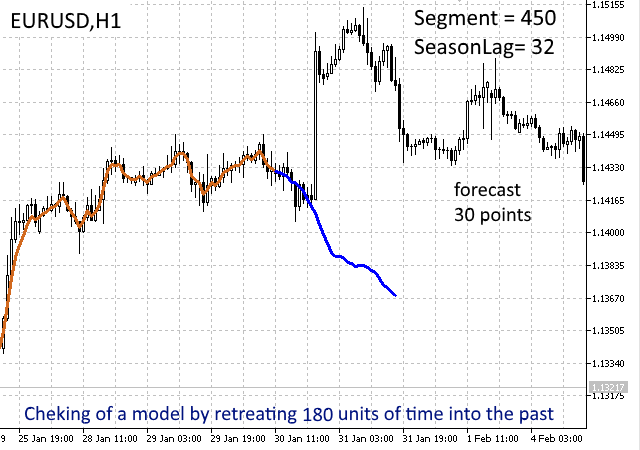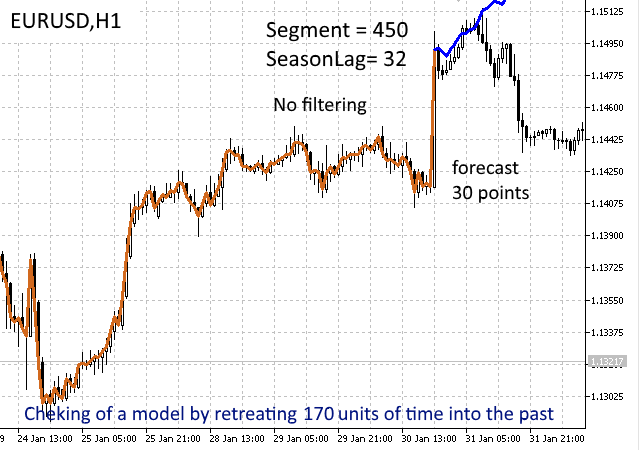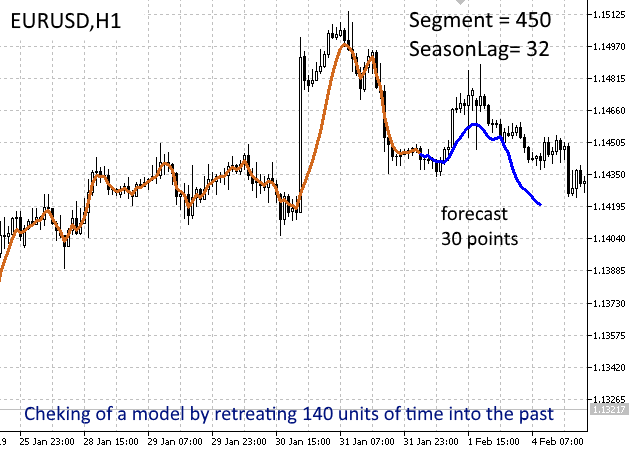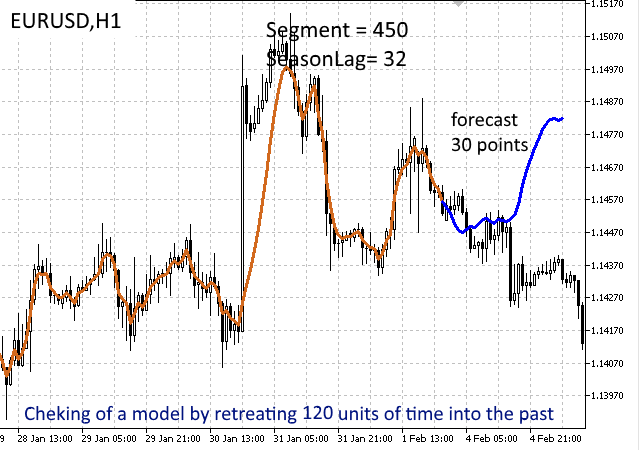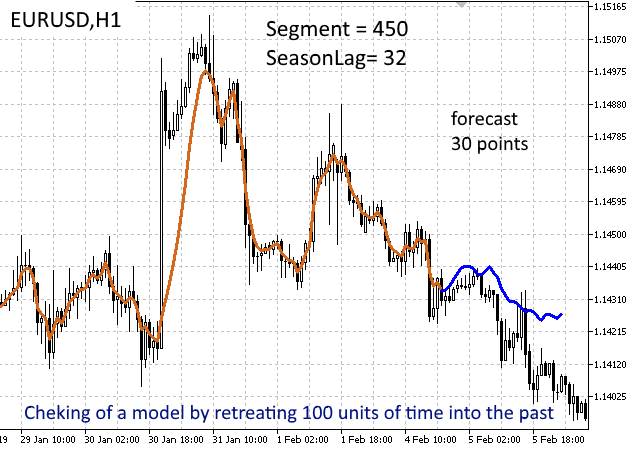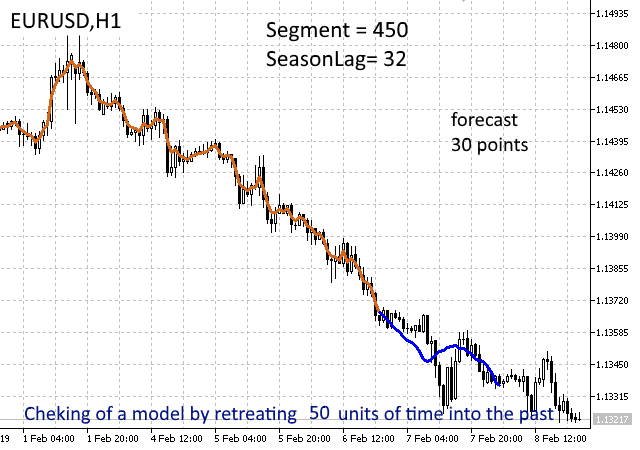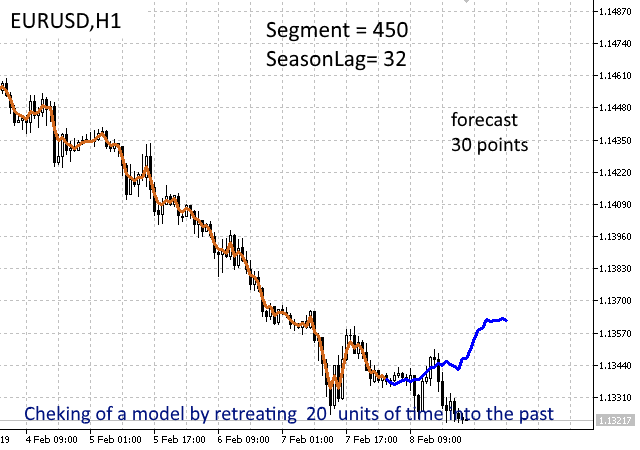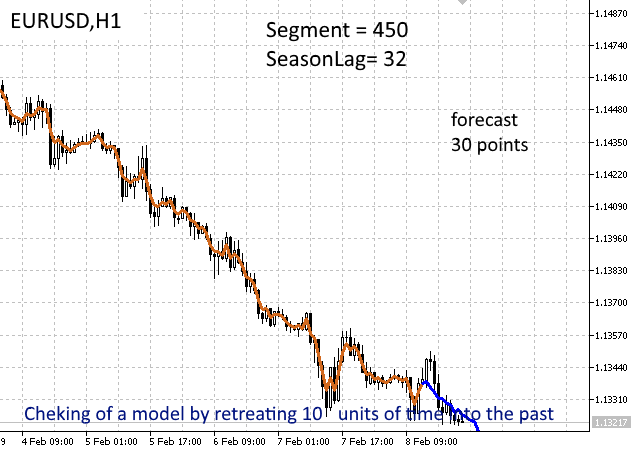### 结论

sarima_plusMCR00.zip 89.16 MB https://pinapfile.com/aKrU7
sarima_plusMCR01.zip 94.75 MB https://pinapfile.com/fvZNS
sarima_plusMCR02.zip 94.76 MB https://pinapfile.com/k7wB5
sarima_plusMCR03.zip 94.76 MB https://pinapfile.com/jwehs
sarima_plusMCR04.zip 94.76 MB https://pinapfile.com/dv8vK
sarima_plusMCR05.zip 94.76 MB https://pinapfile.com/hueKe
sarima_plusMCR06.zip 94.76 MB https://pinapfile.com/c4qzo
sarima_plusMCR07.zip 94.76 MB https://pinapfile.com/eeCkr
sarima_plusMCR08.zip 94.76 MB https://pinapfile.com/jDKTS
sarima_plusMCR09.zip 94.76 MB https://pinapfile.com/dZDJM

MatlabSArima.zip (15634.48 KB)
AdapterSARIMA.zip (3244.29 KB)
ISArimaForecast.mq5 (15.72 KB)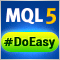用于轻松快速开发 MetaTrader 程序的函数库(第三部分)。 市价订单和仓位的集合，搜索和排序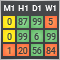作为技术分析工具的 MTF 指标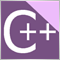10 分钟掌握 MQL5 的 DLL(第二部分)：使用 Visual Studio 2017 创建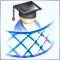开发一个跨平台网格 EA